# Introduction to TBTK – A software development kit for quantum mechanical calculations.

Most recent TBTK release at the time of writing: v0.9.6

## What is TBTK?

As industry is approaching the nanometer scale and quantum mechanical effects begin to play a major role in device manufacturing, it is important to enable engineers to model devices using a quantum mechanical description. Decades of development have lead to a range of algorithms being developed in the scientific community, but most packages used for scientific calculations require extensive experience to handle. Many current scientific packages effectively act as black boxes that present themselves to the user through an interface consisting of a set of input and output files. Moreover, these input and output files are often application specific and therefore severely limits the ease with which applications can be interfaced with each other.

TBTK is first and foremost a C++ library of efficient general purpose data structures that are useful when developing quantum mechanical calculations. The data structures provide a high level of abstraction that allow developers to put more focus on physics and less focus on numerical details without sacrificing performance. The data structures are also meant to enable sharing between different applications and making it possible to grow an echo system of applications for quantum mechanical calculations.

While data structures are at the core of TBTK, it also comes with a range of implemented algorithms such as diagonalization, Arnoldi iteration, Chebyshev expansion of the Green’s function, etc. As such, it is already out of the box ready to be used to setup and perform many types of quantum mechanical calculations. The range of solution methods will certainly continue to expand, but TBTK does not necessarily aim to replace many of the already well developed packages in the scientific community. Instead it aims to simplify the development of new algorithms as well as front ends and back ends for already existing packages. Such front ends and back ends would allow already well established packages to communicate with both each other and new algorithms much more closely. It would also limit the demand on developers to understand the intricate details of specific packages in order to use them and thereby make them accessible to a much larger community of developers.

TBTK already provides partial front ends for libraries such as ARPACK, BLAS, FFTW3, LAPACK, and SuperLU, making it simple for users to utilize the power of these libraries without requiring extensive understanding of them. TBTK also uses the CMake build system to simplify the build procedure, which allows developers to focus on their code rather than struggling with configuring compilation and linking dependencies.

## What does TBTK stand for?

TBTK stands for Tight-Binding ToolKit and reflects the codes origin as a package for performing tight-binding calculations. However, the code has since expanded significantly in scope and is today most appropriately thought of as a package for setting up and solving Hamiltonians on a second-quantized form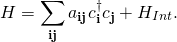Drawing on its tight-binding origin, the coefficients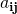are referred to as hopping amplitudes.

## How do I specify a model using TBTK?

Assume we have a model on a square latticewhere angle brackets denote summation over nearest neighbors. We further write the two-dimensional indices as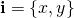and identify the coefficients above asand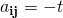. Specifying the model is now a matter of feeding the hopping amplitudesto the application, which is done in TBTK by creating hopping amplitudes using the notation

HoppingAmplitude(value, i, j)

and feeding them to a Model object. For example, we can setup the Hamiltonian above on a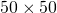square lattice with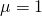and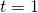as follows

const int SIZE_X = 50;
const int SIZE_Y = 50;
const double mu = 1;
const double t = 1;

Model model;
for(int x = 0; x < SIZE_X; x++){
for(int y = 0; y < SIZE_Y; y++){
model << HoppingAmplitude(-mu, {x, y}, {x, y});

//Add hopping parameter t. The if-statements guard against
//adding terms at the boundary, while "+ HC" ensures that
//the Hermitian conjugate is added too.
if(x+1 < SIZE_X)
model << HoppingAmplitude(-t, {x+1, y}, {x, y}) + HC;
if(y+1 < SIZE_Y)
model << HoppingAmplitude(-t, {x, y+1}, {x, y}) + HC;
}
}
//Construct Hilbert space basis for the model.
model.construct();


Rather than making the chemical potential enter into the problem through the Hamiltonian, it is also possible to instead set it as a single parameter of the model using

model.setChemicalPotential(mu);


Similarly, the temperature and particle statistics can be set using

model.setTemperature(300);
model.setStatistics(Statistics::FermiDirac);
model.setStatistics(Statistics::BoseEinstein);


## How do I choose a method with which to solve my model?

Once a Model object has been specified we can chose solution algorithm by selecting a solver. We can for example chose to solve the model above through diagonalization as follows

Solver::Diagonalizer solver;
solver.setModel(model);
solver.run();


## How do I extract properties from my model?

Once a solver has been chosen, properties are most easily extracted from the model by wrapping the solver in a property extractor. In particular, wrapping the solver in a property extractor often allows us to write code that is independent from a particular solver. The solution method can therefore often be changed later without modifying more than a few lines of code. Using a property extractor we can for example calculate the density of states (DOS) for the model above, in the energy window [-10,10] and using an energy resolution of 1000 points, as follows

PropertyExtractor::Diagonalizer propertyExtractor(solver);
propertyExtractor.setEnergyWindow(-10, 10, 1000);
Property::DOS dos = propertyExtractor.calculateDOS();


It is now possible to for example access the 100th DOS value using

dos(100);


Other properties are calculated for specific indices and it is then similarly possible to access these values using

//Property with index structure.
property0({x, y});
//Property with index and energy structure.
property1({x, y}, 100);


## What solvers are available by default?

In the latest release (v0.9.6) there are four production ready solvers.

### Diagonalizer

Solves the Hamiltonian using diagonalization.

### BlockDiagonalizer

Solves the Hamiltonian utilizing its block structure to reduce the computational cost.

### ArnoldiSolver

Calculates a few eigenvalues and eigenvectors using Arnoldi iteration.

### ChebyshevExpander

Calculates the Green’s function using a Chebyshev expansion method that allows for large systems to be handled on CUDA enabled GPUs.

## What properties are available by default?

In the latest release (v0.9.6) there are seven production ready properties. These are: DOS, Density, EigenValues, LDOS, Magnetization, SpinPolarizedLDOS, and WaveFunction.